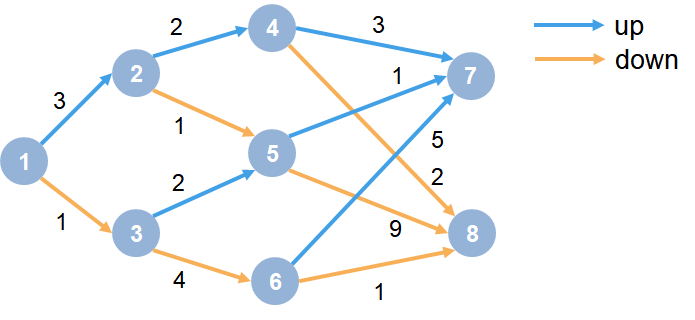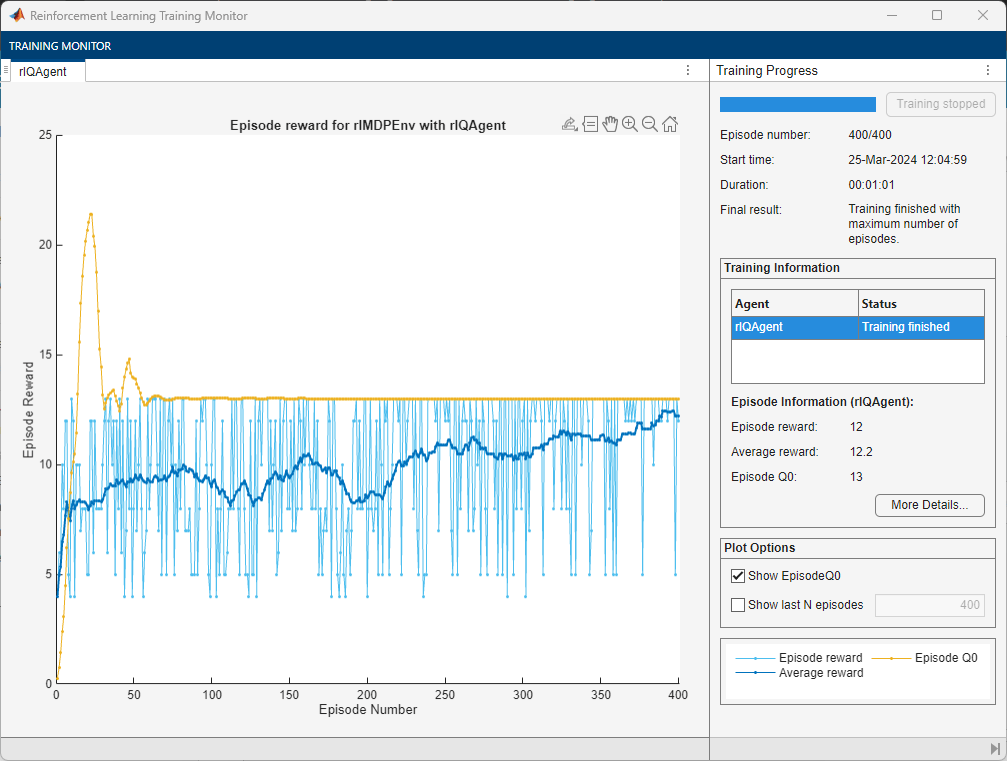# Train Reinforcement Learning Agent in MDP Environment

This example shows how to train a Q-learning agent to solve a generic Markov decision process (MDP) environment. For more information on these agents, see Q-Learning Agents.

The MDP environment has the following graph.Here:

1. Each circle represents a state.

2. At each state there is a decision to go up or down.

3. The agent begins from state 1.

4. The agent receives a reward equal to the value on each transition in the graph.

5. The training goal is to collect the maximum cumulative reward.

### Create MDP Environment

Create an MDP model with eight states and two actions ("up" and "down").

`MDP = createMDP(8,["up";"down"]);`

To model the transitions from the above graph, modify the state transition matrix and reward matrix of the MDP. By default, these matrices contain zeros. For more information on creating an MDP model and the properties of an MDP object, see `createMDP`.

Specify the state transition and reward matrices for the MDP. For example, in the following commands:

• The first two lines specify the transition from state 1 to state 2 by taking action `1` ("up") and a reward of +3 for this transition.

• The next two lines specify the transition from state 1 to state 3 by taking action `2` ("down") and a reward of +1 for this transition.

```MDP.T(1,2,1) = 1; MDP.R(1,2,1) = 3; MDP.T(1,3,2) = 1; MDP.R(1,3,2) = 1;```

Similarly, specify the state transitions and rewards for the remaining rules in the graph.

```% State 2 transition and reward MDP.T(2,4,1) = 1; MDP.R(2,4,1) = 2; MDP.T(2,5,2) = 1; MDP.R(2,5,2) = 1; % State 3 transition and reward MDP.T(3,5,1) = 1; MDP.R(3,5,1) = 2; MDP.T(3,6,2) = 1; MDP.R(3,6,2) = 4; % State 4 transition and reward MDP.T(4,7,1) = 1; MDP.R(4,7,1) = 3; MDP.T(4,8,2) = 1; MDP.R(4,8,2) = 2; % State 5 transition and reward MDP.T(5,7,1) = 1; MDP.R(5,7,1) = 1; MDP.T(5,8,2) = 1; MDP.R(5,8,2) = 9; % State 6 transition and reward MDP.T(6,7,1) = 1; MDP.R(6,7,1) = 5; MDP.T(6,8,2) = 1; MDP.R(6,8,2) = 1; % State 7 transition and reward MDP.T(7,7,1) = 1; MDP.R(7,7,1) = 0; MDP.T(7,7,2) = 1; MDP.R(7,7,2) = 0; % State 8 transition and reward MDP.T(8,8,1) = 1; MDP.R(8,8,1) = 0; MDP.T(8,8,2) = 1; MDP.R(8,8,2) = 0;```

Specify states `"s7"` and `"s8"` as terminal states of the MDP.

`MDP.TerminalStates = ["s7";"s8"];`

Create the reinforcement learning MDP environment for this process model.

`env = rlMDPEnv(MDP);`

To specify that the initial state of the agent is always state 1, specify a reset function that returns the initial agent state. This function is called at the start of each training episode and simulation. Create an anonymous function handle that sets the initial state to 1.

`env.ResetFcn = @() 1;`

Fix the random generator seed for reproducibility.

`rng(0)`

### Create Q-Learning Agent

To create a Q-learning agent, first create a Q table using the observation and action specifications from the MDP environment. Set the learning rate of the representation to `1`.

```obsInfo = getObservationInfo(env); actInfo = getActionInfo(env); qTable = rlTable(obsInfo, actInfo); qRepresentation = rlQValueRepresentation(qTable, obsInfo, actInfo); qRepresentation.Options.LearnRate = 1;```

Next, create a Q-learning agent using this table representation, configuring the epsilon-greedy exploration. For more information on creating Q-learning agents, see `rlQAgent` and `rlQAgentOptions`.

```agentOpts = rlQAgentOptions; agentOpts.DiscountFactor = 1; agentOpts.EpsilonGreedyExploration.Epsilon = 0.9; agentOpts.EpsilonGreedyExploration.EpsilonDecay = 0.01; qAgent = rlQAgent(qRepresentation,agentOpts); %#ok<NASGU> ```

### Train Q-Learning Agent

To train the agent, first specify the training options. For this example, use the following options:

• Train for at most 500 episodes, with each episode lasting at most 50 time steps.

• Stop training when the agent receives an average cumulative reward greater than 10 over 30 consecutive episodes.

For more information, see `rlTrainingOptions`.

```trainOpts = rlTrainingOptions; trainOpts.MaxStepsPerEpisode = 50; trainOpts.MaxEpisodes = 500; trainOpts.StopTrainingCriteria = "AverageReward"; trainOpts.StopTrainingValue = 13; trainOpts.ScoreAveragingWindowLength = 30;```

Train the agent using the `train` function. This may take several minutes to complete. To save time while running this example, load a pretrained agent by setting `doTraining` to `false`. To train the agent yourself, set `doTraining` to `true`.

```doTraining = false; if doTraining % Train the agent. trainingStats = train(qAgent,env,trainOpts); %#ok<UNRCH> else % Load pretrained agent for the example. load('genericMDPQAgent.mat','qAgent'); end```### Validate Q-Learning Results

To validate the training results, simulate the agent in the training environment using the `sim` function. The agent successfully finds the optimal path which results in cumulative reward of `13`.

```Data = sim(qAgent,env); cumulativeReward = sum(Data.Reward)```
```cumulativeReward = 13 ```

Since the discount factor is set to `1`, the values in the Q table of the trained agent match the undiscounted returns of the environment.

```QTable = getLearnableParameters(getCritic(qAgent)); QTable{1}```
```ans = 8×2 12.9874 7.0759 -7.6425 9.9990 10.7193 0.9090 5.9128 -2.2466 6.7830 8.9988 7.5928 -5.5053 0 0 0 0 ```
`TrueTableValues = [13,12;5,10;11,9;3,2;1,9;5,1;0,0;0,0]`
```TrueTableValues = 8×2 13 12 5 10 11 9 3 2 1 9 5 1 0 0 0 0 ```